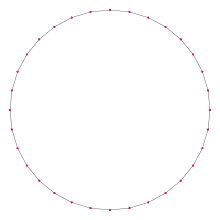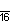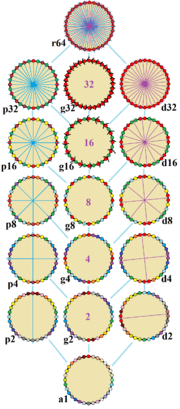Type Regular polygon
Edges and vertices 32
Schläfli symbol {32}, t{16}, tt{8}, ttt{4}
Coxeter diagramSymmetry group Dihedral (D32), order 2×32
Internal angle (degrees) 168.75°
Dual polygon Self
Properties Convex, cyclic, equilateral, isogonal, isotoxal

In geometry, a triacontadigon (or triacontakaidigon) or 32-gon is a thirty-two-sided polygon. In Greek, the prefix triaconta- means 30 and di- means 2. The sum of any triacontadigon's interior angles is 5400 degrees.

An older name is tricontadoagon. Another name is icosidodecagon, suggesting a (20 and 12)-gon, in parallel to the 32-faced icosidodecahedron, which has 20 triangles and 12 pentagons.

The regular triacontadigon can be constructed as a truncated hexadecagon, t{16}, a twice-truncated octagon, tt{8}, and a thrice-truncated square. A truncated triacontadigon, t{32}, is a hexacontatetragon, {64}.

One interior angle in a regular triacontadigon is 16814°, meaning that one exterior angle would be 1114°.

The area of a regular triacontadigon is (with t = edge length)### Construction

As 32 = 25 (a power of two), the regular triacontadigon is a constructible polygon. It can be constructed by an edge-bisection of a regular hexadecagon.

## SymmetryThe symmetries of a regular triacontadigon. Lines of reflections are blue through vertices, and purple through edges. Gyrations are given as numbers in the center. Vertices are colored by their symmetry positions.

The regular triacontadigon has Dih32 dihedral symmetry, order 64, represented by 32 lines of reflection. Dih32 has 5 dihedral subgroups: Dih16, Dih8, Dih4, Dih2 and Dih1 and 6 more cyclic symmetries: Z32, Z16, Z8, Z4, Z2, and Z1, with Zn representing π/n radian rotational symmetry.

On the regular triacontadigon, there are 17 distinct symmetries. John Conway labels these lower symmetries with a letter and order of the symmetry follows the letter. He gives r64 for the full reflective symmetry, Dih16, and a1 for no symmetry. He gives d (diagonal) with mirror lines through vertices, p with mirror lines through edges (perpendicular), i with mirror lines through both vertices and edges, and g for rotational symmetry. a1 labels no symmetry.

These lower symmetries allows degrees of freedoms in defining irregular triacontadigons. Only the g32 subgroup has no degrees of freedom but can seen as directed edges.